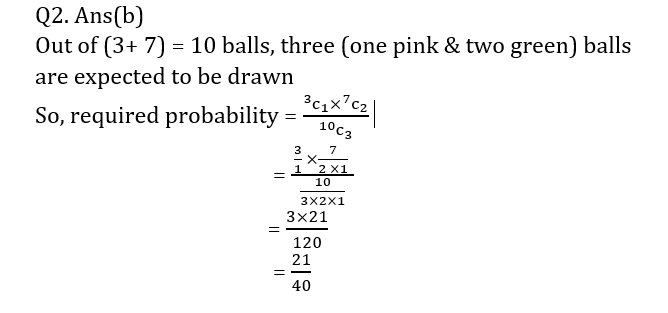# Sampling Methods: Caiib Paper 1 (Module B), Unit 2

## Sampling Methods: Caiib Paper 1 (Module B), Unit 2

Dear Bankers,
We all know that CAIIB exams are conducted by the Indian Institute of Banking and Finance (IIBF).  CAIIB is said to be one of the difficult courses to be cleared for the bankers. But we assure you that with the help of our “CAIIB study material”, you will definitely clear the CAIIB exam.
CAIIB exams are conducted twice in a year. Candidates should have completed JAIIB before appearing for CAIIB Exam. Here, we will provide detailed notes of every unit of the CAIIB Exam on the latest pattern of IIBF.
So, here we are providing “Unit 2: Sampling Methods of “Module B: Business Mathematics” from “Paper 1: Advanced Bank Management (ABM)”.

The Article is Caiib Unit 2: Sampling Methods

### Sampling

• Sampling is a process used in statistical analysis in which a predetermined number of observations are taken from a larger population. The methodology used to sample from a larger population depends on the type of analysis being performed.
##### Types of sampling

There are two methods of selecting from populations

• Non- random or judgement sampling
• Random or probability sampling

### Random Sampling

• A probability sampling method is any method of sampling that utilizes some form of random selection. In order to have a random selection method, you must set up some process or procedure that assures that the different units in your population have equal probabilities of being chosen.
##### Type of Random Sampling

There are four main type of random sampling

1. Simple Random Sampling (SRS)
2. Stratified Sampling
3. Cluster Sampling
4. Systematic Sampling
• Simple Random Sampling (SRS): Simple Random Sampling selects samples by methods that allow each possible sample to have an equal probability of being picked and each item in the entire population to have an equal chance or being included in the sample.
• Systematic Sampling: In systematic sampling, elements are selected from the population at a uniform level that is measured in time, order, or space. If we wanted to interview every twentieth student on a college campus, we would choose a random starting point in the first twenty names in the student directory and then pick every twentieth name thereafter.
• Stratified Sampling: To use stratified sampling, we divide the population into relatively homogenous groups, called strata. Then we use one of two approaches. Either we select at random from each stratum a specified number of elements corresponding to the proportion of that stratum in the population as a whole or we draw an equal number of elements from each stratum and give weight to the results according to the stratum’s proportion of total population.
• Cluster Sampling: In cluster sampling, we divide the population into groups or clusters and then select a random sample of these clusters. We assume that these individual clusters are representative of the population as a whole. If a market Research team is attempting to determine by sampling the average number of television sets per household in a large city, they could use a city map and divide the territory into blocks and then choose a certain number of blocks (clusters) for interviewing. Every household in each of these blocks would be interviewed. A well designed cluster sampling procedure can produce a more precise sample at considerably less cost than that of simple random sampling.
##### Standard Error
• Standard deviation of the distribution of the sample means is called the standard error of the mean.

#### Numerical on Sampling

Q1. A sack contains 3 pink balls and 7 green balls. What is probability to draw one pink ball and two green balls in one draw?

(a)23/40

(b)21/40

(c)27/40

(d)20/39Q2.A sack contains 4 black balls 5 red balls. What is probability to draw 1 black ball and 2 red balls in one draw?

(a) 11/19

(b) 10/21

(c)12/22

(d) 19/11

Ans: B

Solution :

Out of 9, 3 (1 black & 2 red) are expected to be drawn)

Hence sample space

n(S) = 9c3

= 9!/(6!×3!)

= 362880/4320

= 84

Now out of 4 black ball 1 is expected to be drawn hence

n(B) = 4c1

= 4

Same way out of 5 red balls 2 are expected be drawn hence

n(R) = 5c2

= 5!/(3!×2!)

= 120/12

= 10

Then P(B U R) = n(B)×n(R)/n(S)

i.e 4×10/84 = 10/21

#### Read More Article Caiib Paper-1, Module A

Visit Online Mock test Site: test.ambitiousbaba.com